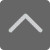# 小学口算题大全：二年级100以内加减法口算题（五）

2018-11-02 16:36:41 　来源：网络 文章作者：网络
 免费下载 3个G小报手抄报电子版 小学1-5年级上册期末复习资料 [ 戳我免费下载 ]

40+29+1=

62+15=

54-13=

84+3+7=

68-60=

16+13=

86+4+6=

77-48=

11+17=

46+23+17=

84-1=

18+20=

22+45+15=

61-23=

62+28=

0+55+5=

13+69=

92-3=

77+22+8=

53+18=

20+22=

54+40+10=

89-81=

55+20=

23+25+15=加入QQ群，与更多家长交流经验！
2
 小学交流群：639215153 初中交流群：551802726 高中交流群：591337342 上海幼儿园升小学家长学习交流群：772707735